# Modelling the number of reverse dependencies

#### 2020-08-10

From the dependency network of all CRAN packages, we have seen that reverse dependencies of all CRAN packages seem to follow the power law. This echoes the phenomenon in other software dependencies observed by, for example, LaBelle and Wallingford, 2004, Baxter et al., 2006, Jenkins and Kirk, 2007, Wu et al. 2007, Louridas et al., 2008, Zheng et al., 2008, Kohring, 2009, Li et al., 2013, Bavota et al. 2015, and Cox et al., 2015. In this vignette, we will fit the discrete power law to model this number of reverse dependencies, using functions with the suffix upp, namely dupp(), Supp() and mcmc_upp(). While we shall focus on “Imports”, which is one of the serveral kinds of dependencies in R, the same analysis can be carried out for all other types.

library(crandep)
library(igraph)
library(dplyr)
library(ggplot2)

## Obtaining the reverse dependencies

In the vignette on all CRAN pacakges, we looked at the dependency type Depends. Here, we look at the dependency type Imports, using the same workflow. As we are using the forward dependencies to construct the network, the number of reverse dependencies is equivalent to the in-degree of a package, which can be obtained via the function igraph::degree(). We then construct a data frame to hold this in-degree information.

g0.imports <- get_graph_all_packages(type = "imports")
d0.imports <- g0.imports %>% igraph::degree(mode = "in")
df0.imports <-
data.frame(name = names(d0.imports), degree = as.integer(d0.imports)) %>%
dplyr::arrange(dplyr::desc(degree), name)
#>        name degree
#> 1      Rcpp   1817
#> 2   ggplot2   1755
#> 3     dplyr   1649
#> 4      MASS   1108
#> 5  magrittr   1092
#> 6   stringr    923
#> 7     rlang    849
#> 8    tibble    844
#> 9  jsonlite    770
#> 10    tidyr    735

For the purpose of verification, we use the reverse dependencies to construct the network, then look at the out-degrees of the packages this time.

g0.rev_imports <- get_graph_all_packages(type = "reverse imports")
d0.rev_imports <- g0.rev_imports %>% igraph::degree(mode = "out") # note the difference to above
df0.rev_imports <-
data.frame(name = names(d0.rev_imports), degree = as.integer(d0.rev_imports)) %>%
dplyr::arrange(dplyr::desc(degree), name)
#>        name degree
#> 1      Rcpp   1817
#> 2   ggplot2   1755
#> 3     dplyr   1649
#> 4      MASS   1108
#> 5  magrittr   1092
#> 6   stringr    923
#> 7     rlang    849
#> 8    tibble    844
#> 9  jsonlite    770
#> 10    tidyr    735

Theoretically, the two data frames are identical. Any possible (but small) difference is due to the CRAN pages being updated while scraping.

identical(df0.imports, df0.rev_imports)
#>  TRUE
setdiff(df0.imports, df0.rev_imports)
#>  name   degree
#> <0 rows> (or 0-length row.names)
setdiff(df0.rev_imports, df0.imports)
#>  name   degree
#> <0 rows> (or 0-length row.names)

## Exploratory analysis and selecting the threshold

We construct a data frame for the empirical frequencies and survival function at the whole range of data.

df1.imports <- df0.imports %>%
dplyr::filter(degree > 0L) %>% # to prevent warning when plotting on log-log scale
dplyr::count(degree, name = "frequency") %>%
dplyr::arrange(dplyr::desc(degree)) %>%
dplyr::mutate(survival = cumsum(frequency)/sum(frequency))

Before fitting the discrete power law, to be determined first is a threshold above which it is appropriate. We will visualise the degree distribution to determine such threshold.

gg0 <- df1.imports %>%
ggplot2::ggplot() +
ggplot2::geom_point(aes(degree, frequency), size = 0.75) +
ggplot2::scale_x_log10() +
ggplot2::scale_y_log10() +
ggplot2::coord_cartesian(ylim = c(1L, 1e+3L)) +
ggplot2::theme_bw(12)
gg0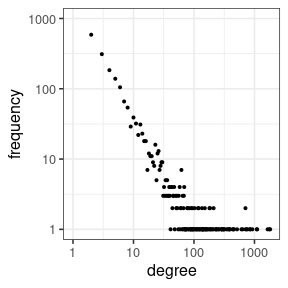The power law seems appropriate for the whole range of data, and so, for illustration purposes, the threshold will be set at 1 inclusive. 0’s will be excluded anyway because, as we will see, the probability mass function (PMF) is not well-defined at 0.

While it is straightforward to determine the threshold here, such linearity over the whole range might not be seen for other data. The package poweRlaw provides functions for and references to more systematic/objective procedures of selecting the threshold.

## Fitting the discrete power law

We use the function mcmc_upp() to fit the discrete power law, of which the PMF is proportional to $$x^{-\alpha}$$, where $$\alpha$$ is the lone scalar parameter. Here we will use the parameter $$\xi_1=1/(\alpha-1)$$ to align with the parameterisation of mcmc_mix() and other distributions in extreme value theory, which is an extension of the power law.

The Bayesian approach is used here for inference, meaning that a prior has to be set for the parameters. We assume a uniform distribution $$U(a_{\xi_1}=0, b_{\xi_1}=100)$$ for $$\xi_1$$. Markov chain Monte Carlo (MCMC) is used as the inference algorithm.

x <- dplyr::filter(df0.imports, degree > 0L)$degree # data u <- 1L # threshold xi1 <- 1.0 # initial value a_xi1 <- 0.0 # lower bound of uniform distribution b_xi1 <- 100.0 # upper bound of uniform distribution set.seed(3075L) mcmc0.imports <- mcmc_upp(x = x, u = u, xi1 = xi1, a_xi1 = a_xi1, b_xi1 = b_xi1) # takes seconds Now we have the samples representing the posterior distribution of $$\xi_1$$: mcmc0.imports %>% ggplot2::ggplot() + ggplot2::geom_density(aes(xi1)) + ggplot2::theme_bw(12)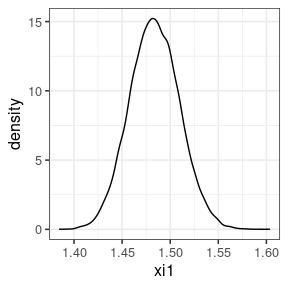We can obtain the resulting posterior of $$\alpha=1/\xi_1+1$$. mcmc0.imports <- mcmc0.imports %>% dplyr::mutate(alpha = 1.0 / xi1 + 1.0) mcmc0.imports %>% ggplot2::ggplot() + ggplot2::geom_density(aes(alpha)) + ggplot2::theme_bw(12)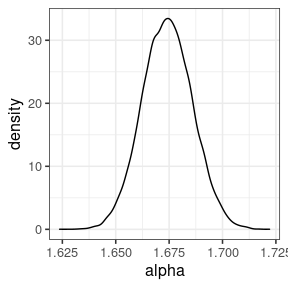This means the number of reverse “Imports” follows approximately a power law with exponent 1.67. We can also calculate the fitted frequencies and survival function, using dupp() and Supp() respectively. n0 <- sum(df1.imports$frequency) # TOTAL number of data points in x
## or n0 <- length(x)
n1 <- length(df1.imports$frequency) # number of UNIQUE data points N <- length(mcmc0.imports$xi1)
freq0 <- surv0 <- matrix(as.numeric(NA), N, n1)
for (i in seq(N)) {
freq0[i,] <- n0 * dupp(x = df1.imports$degree, u = 1L, xi1 = mcmc0.imports$xi1[i])
surv0[i,] <- Supp(x = df1.imports$degree, u = 1L, xi1 = mcmc0.imports$xi1[i])
}
df1.imports <- df1.imports %>%
dplyr::mutate(
frequency.mean = apply(freq0, 2, mean),
frequency.qlow = apply(freq0, 2, quantile, p = 0.025),
frequency.qupp = apply(freq0, 2, quantile, p = 0.975),
survival.mean = apply(surv0, 2, mean),
survival.qlow = apply(surv0, 2, quantile, p = 0.025),
survival.qupp = apply(surv0, 2, quantile, p = 0.975)
)

Finally, we overlay the fitted line (blue, dashed) and credible intervals (red, dotted) on the plot above to check goodness-of-fit:

gg1 <- df1.imports %>%
ggplot2::ggplot() +
ggplot2::geom_point(aes(degree, frequency), size = 0.75) +
ggplot2::geom_line(aes(degree, frequency.mean), col = 4, lty = 2) +
ggplot2::geom_line(aes(degree, frequency.qlow), col = 2, lty = 3) +
ggplot2::geom_line(aes(degree, frequency.qupp), col = 2, lty = 3) +
ggplot2::scale_x_log10() +
ggplot2::scale_y_log10() +
ggplot2::coord_cartesian(ylim = c(1L, 1e+3L)) +
ggplot2::theme_bw(12)
gg1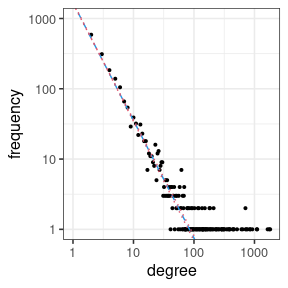The corresponding plot using the survival function can also be obtained:

gg2 <- df1.imports %>%
ggplot2::ggplot() +
ggplot2::geom_point(aes(degree, survival), size = 0.75) +
ggplot2::geom_line(aes(degree, survival.mean), col = 4, lty = 2) +
ggplot2::geom_line(aes(degree, survival.qlow), col = 2, lty = 3) +
ggplot2::geom_line(aes(degree, survival.qupp), col = 2, lty = 3) +
ggplot2::scale_x_log10() +
ggplot2::scale_y_log10() +
ggplot2::theme_bw(12)
gg2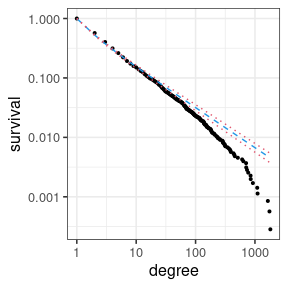This shows the discrete power law doesn’t fit as good as it seems according to the frequency plot. Potential improvements include using a higher threshold, which inevitably means throwing away some data, or using a more flexible distribution, such as those in extreme value theory. Provided in this package for such purposes are dmix(), Smix() and mcmc_mix(), which are functions for discrete extreme value mixture distributions introduced in Lee and Eastoe, 2020.